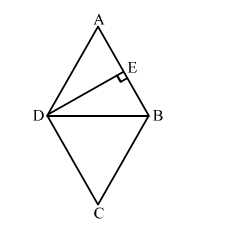# In a rhombus ABCD, the altitude from D to the side AB bisects AB.

Question:

In a rhombus ABCD, the altitude from D to the side AB bisects AB. Find the angles of the rhombus.

Solution:Given: $A B C D$ is a rhombus, $D E$ is altitude which bisects $A B$ i.e. $A E=E B$

In $\Delta A E D$ and $\Delta B E D$,

$D E=D E$        (Common side)

$\angle D E A=\angle D E B=90^{\circ}$     (Given)

$A E=E B$    (Given)

$\therefore \Delta A E D \cong \Delta B E D$   (By SAS congruence Criteria)

$\Rightarrow A D=B D$      (CPCT)

Also, $A D=A B$      (Sides of rhombus are equal)

$\Rightarrow A D=A B=B D$

Thus, $\Delta A B D$ is an equilateral triangle.

Therefore, $\angle A=60^{\circ}$

$\Rightarrow \angle C=\angle A=60^{\circ}$    (Opposite angles of rhombus are equal)

And, $\angle A B C+\angle B C D=180^{\circ}$   (Adjacent angles of rhombus are supplementary.)

$\Rightarrow \angle A B C+60^{\circ}=180^{\circ}$

$\Rightarrow \angle A B C=180^{\circ}-60^{\circ}$

$\Rightarrow \angle A B C=120^{\circ}$

$\Rightarrow \angle A D C=\angle A B C=120^{\circ}$

Hence, the angles of rhombus are $60^{\circ}, 120^{\circ}, 60^{\circ}$ and $120^{\circ}$.